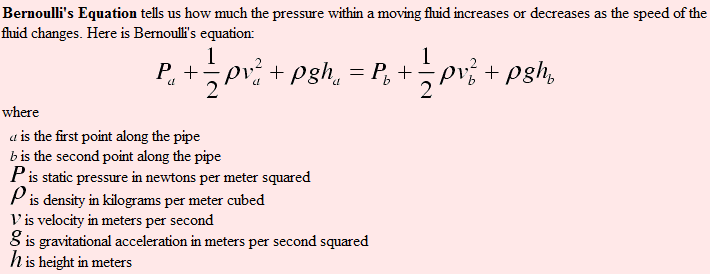# Torricelli's Law

Lil_Aziz1
Hey I have a question on the derivation of Torricelli's Law. Bernoulli's Equation states the following:Now let's say that the left side of the equation is the top of the tank and the right side of the equation is the bottom of the tank. The origin is at the bottom of the tank.

We can say that $$P_a = P_{atm}, v_a \approx 0, h_b = 0$$

Therefore, our equation becomes

$$P_{atm} + pgh = P_{bottom of tank} + 1/2pv^2$$

Now torricelli's law states that $$v = \sqrt{2gh}$$, which we only get if we neglect the difference in pressure. Is that true? When I looked up torricelli's law, they said that the only thing neglected was viscosity and nothing about the change in pressure. What am I doing wrong?

foges
Not that i know much about fluid dynamics, but in assuming that $$v_a =0$$ you are also assuming that the amount of water flowing out of the bottom is negligible in comparison to the tank of water. Most of the water at the bottom of the tank in fact has a velocity $$v_b=0$$ and then the equation adds up. Torricelli's law is only the speed that a minute amount of water would have if you let some flow out at the bottom, not the actual velocity of all the water at the bottom of the tank.

On a second look you can actually derive Torricellis law from the equation $$P_{atm} + pgh = P_{bottom of tank} + 1/2pv^2$$. If you make a small hole in the tank, then the water streaming out of the tank must have a pressure $$P_{atm}$$ as well, then $$P_{atm} + pgh = P_{atm} + 1/2pv^2 \Rightarrow pgh = 1/2pv^2 \Rightarrow v = \sqrt{2gh}$$.

Last edited:
Lil_Aziz1
Oh wow I didn't think about the fact that if there is a hole, the pressure at the bottom of the tank (more specifically, the small hole) is not $$P=pgh$$. Thanks!

Hossein moj
hi
but
if the pressure at the bottom of the tank is equal to air pressure , it means that the pressure at the top of water and at the hole is equal to air pressure. so water cannot move and flow and must remain static.
thanks

IsometricPion
Bernoulli's equation is equivalent to conservation of energy. For any given (ideal) volume of water, the equation must hold at all times. This means that one need not be concerned about how the water leaves the container. So, applying the equation with the "a" label referring to a small volume of water at the top of the container and "b" applying to the same volume of water that has just exited the container a distance h below the level of the water, one has Pa=Pb=PAtm, va≈0 (assuming the volume of water in the container is much larger than the flow rate out of the container) hA-hb=h. After some algebra one obtains Toricelli's law. The pressure at the bottom of the container is greater than the atmospheric pressure due to the depth of the water in the container. After the water leaves the container the water in the container is no longer exerting pressure on it, which is why Pa=Pb. Note that if va=Vb=0, then the Bernoulli equation allows one to derive the pressure at a given depth, ha-hb.

Hossein moj
"After the water leaves the container the water in the container is no longer exerting pressure on it, which is why Pa=Pb."
.............................................................
thank you "IsometricPion"